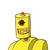# 20A bag contains balls which are numbers from ‘1’ to ’60’. A ball isdrawn at from the bag, the probability that it bea

20
A bag contains balls which are numbers from ‘1’ to ’60’. A ball is
drawn at from the bag, the probability that it bears a two digit
number multiples of 7 ?​

### 1 thought on “20<br />A bag contains balls which are numbers from ‘1’ to ’60’. A ball is<br />drawn at from the bag, the probability that it bea”

1.probabilyti is 7/60

Step-by-step explanation:

no. of ball n(s) = 60

balls with multiple of 7 and bears two digit number

(14,21,28,35,42,49,56)

no. of twoo digits balls n(a) =7

probability =n(a) /n(a) =7/60